# How to calculate average speed from distance and time in Excel?

In a typical scenario, we are able to easily calculate the average speed in an Excel worksheet by using the formula Speed = Distance / Hours. However, there are situations in which the hours are not represented by numbers but rather a time format, how would you handle this work in Excel?

In this tutorial, we will use a simple formula to calculate the average speed based on the distance travelled and the amount of time spent doing so.

Let’s understand step by step with an example.

### Step 1

In the first step, let us assume we have a sample data for calculating average speed from distance and time as shown in the below screenshot for the same.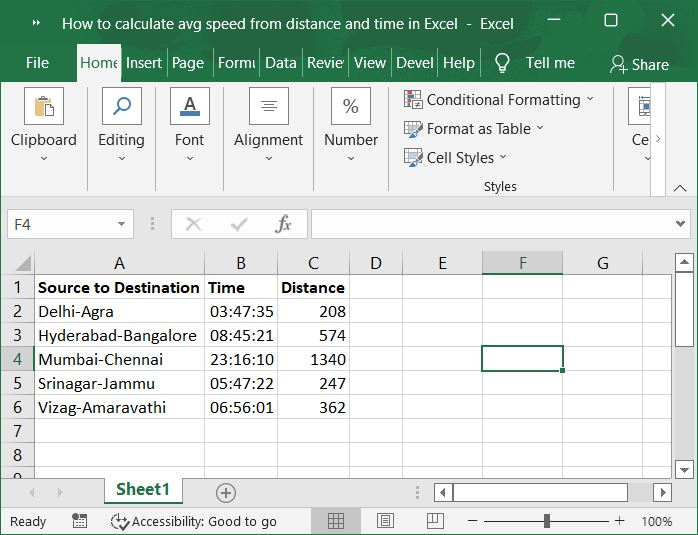### Step 2

Simply insert this formula into a blank cell located in the area of the spreadsheet where you wish the result to appear. Please refer to the below screenshot for the same.

### Formula

=C2/(B2*24)


Important Note − Please adjust the values of C2 and B2 in the formula that was just presented, these are the distance and the amount of time, respectively.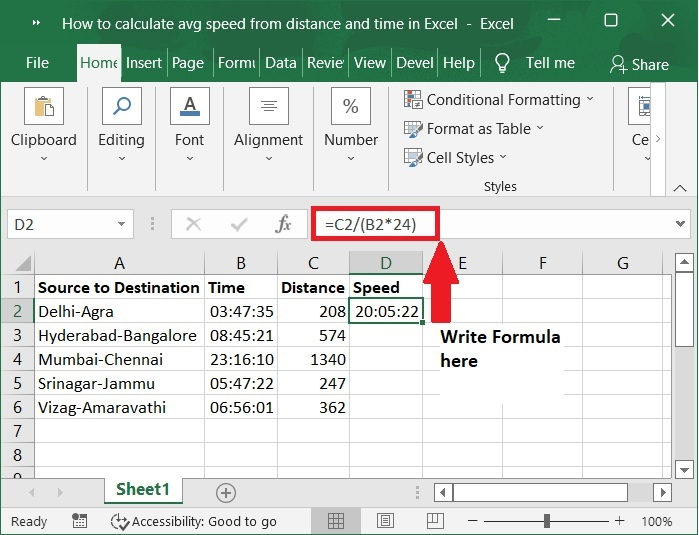### Step 3

After that, drag the fill handle all the way down to the cells where you want the results to appear. Please check out the below screenshot for the same.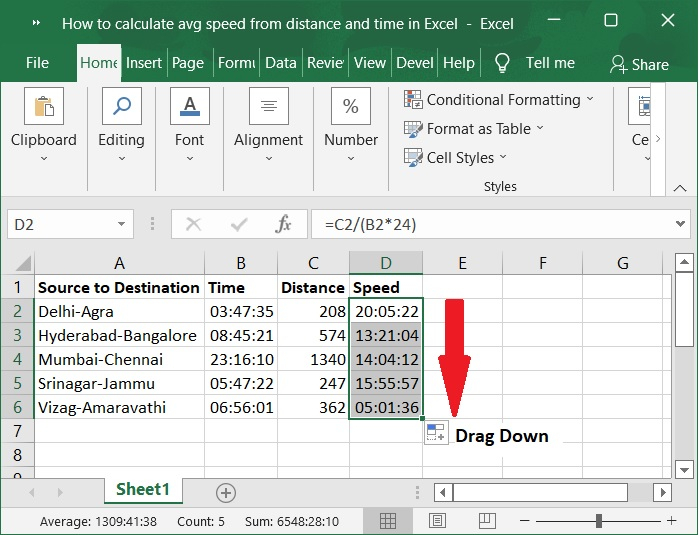### Step 4

After that, you should convert the results from the time format to the general format by selecting General from the drop-down list located in the Number group of the Home tab. Please refer to the below screenshot for the same.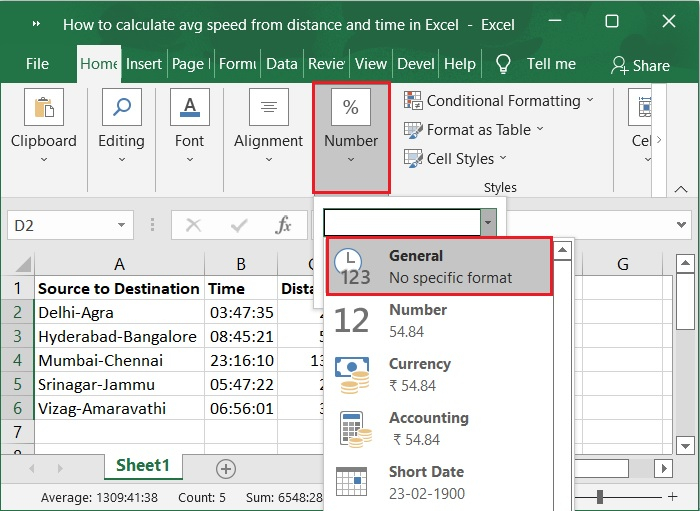### Step 5

Then you will obtain the accurate results that meet your requirements. Please check out below screenshot for the same.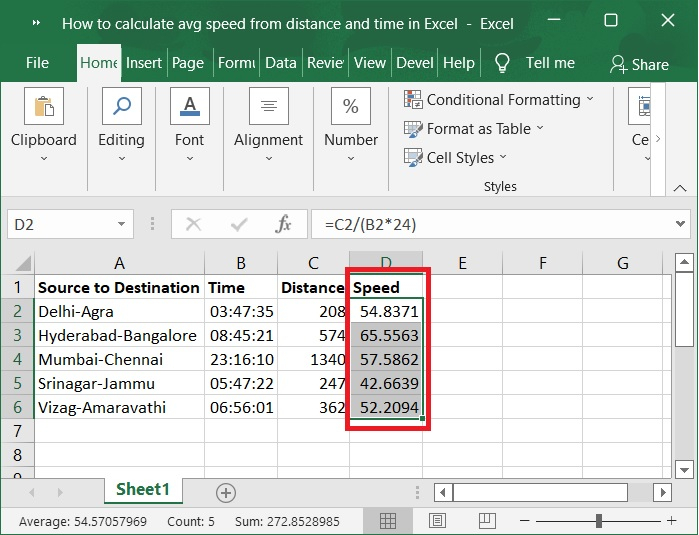Important Note − Use the following formula to figure out the average speed if you have two different lists of start time and end time to compare.

### Formula

=B2/(24*(C2-D2))


Here B2 represents Distance, C2 represents Start time and D2 represents End time.

## Conclusion

In this tutorial, we explained how you can calculate the average speed from distance and time by using formulas in Excel.

Updated on: 03-Feb-2023

2K+ Views